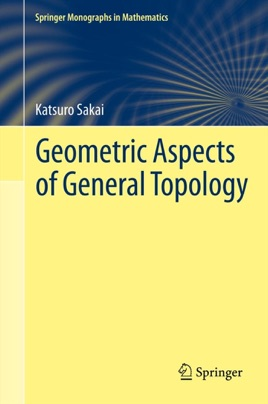• 94,99 €

## Description de l’éditeur

This book is designed for graduate students to acquire knowledge of dimension theory, ANR theory (theory of retracts), and related topics. These two theories are connected with various fields in geometric topology and in general topology as well. Hence, for students who wish to research subjects in general and geometric topology, understanding these theories will be valuable. Many proofs are illustrated by figures or diagrams, making it easier to understand the ideas of those proofs. Although exercises as such are not included, some results are given with only a sketch of their proofs. Completing the proofs in detail provides good exercise and training for graduate students and will be useful in graduate classes or seminars.

Researchers should also find this book very helpful, because it contains many subjects that are not presented in usual textbooks, e.g., dim X × I = dim X + 1 for a metrizable space X; the difference between the small and large inductive dimensions; a hereditarily infinite-dimensional space; the ANR-ness of locally contractible countable-dimensional metrizable spaces; an infinite-dimensional space with finite cohomological dimension; a dimension raising cell-like map; and a non-AR metric linear space. The final chapter enables students to understand how deeply related the two theories are.

Simplicial complexes are very useful in topology and are indispensable for studying the theories of both dimension and ANRs. There are many textbooks from which some knowledge of these subjects can be obtained, but no textbook discusses non-locally finite simplicial complexes in detail. So, when we encounter them, we have to refer to the original papers. For instance, J.H.C. Whitehead's theorem on small subdivisions is very important, but its proof cannot be found in any textbook. The homotopy type of simplicial complexes is discussed in textbooks on algebraic topology using CW complexes, but geometrical arguments using simplicial complexes are rather easy.